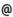## "Babes-Bolyai" University of Cluj-Napoca Faculty of Mathematics and Computer Science

 Analysis and complexity of algorithms
 Code Semes-ter Hours: C+S+L Credits Type Section MI019 6 2+1+0 5 optional Informatică
 Teaching Staff in Charge
 Lect. IONESCU Clara, Ph.D., claracs.ubbcluj.ro
 Aims After studying the subjects presented in the lectures, the students will be able to: * use mechanisms for algorithms analysis * perform complexity analysis for algorithms * identify the class of a given problem * design and analyze algorithms * design programs according to the users' requests * to check the correctness of a software product after building it * to use developing tools for applications
 Content Introduction (Algorithms. Algorithm analysis. Algorithm design) * Mechanisms for complexity analysis (Asymptotic notation. Standard notations and common functions. Amortized analysis) * Mathematical foundations (Summations. Recurrences and recursion. Sets. Counting and probability) * Number-theoretic algorithms * Combinatorial algorithms * Dirichlet's box * Classifying problems according to their time complexity. NP-Completeness * Programming techniques (Divide et Impera. Sorting. Searching. String matching) * Advanced programming techniques (Greedy algorithms. Heuristic greedy algorithms. Dynamic programming. Elements of computational geometry) * Solving NP-complete problems and comparison of different solutions (Backtracking. Heuristic greedy algorithms. Probabilistic algorithms. Approximation algorithms. Evolutionary algorithms)
 References 1. CORMEN, T. - LEISERSON, CH. - RIVEST, R. - STEIN, C. : Új algoritmusok, Scolar Kiadó, Budapest, 2003. 2. CORMEN, T. - LEISERSON, CH. - RIVEST, R. : Introducere în algoritmi, Editura Computer Libris Agora, Cluj-Napoca, 2000. 3. BRASSARD, G. - BRATLEY, P. : Algorithmics: Theory and Practice, Prentice Hall, 1988. 4. BRASSARD, G. - BRATLEY, P. : Fundamentals of Algorithmics, Prentice Hall, 1996. 5. HOROWITZ, E. - SAHNI, S. : Fundamentals of Computer Algorithms, Computer Science Press, 1978. 6. HOROWITZ, E. - SAHNI, S. - RAJASEKARAN, S. : Computer Algorithms, Computer Science Press, 1998. 7. KNUTH, D. : Arta programării calculatoarelor, vol. III, Sortare şi Căutare, Editura Teora, Bucuresti, 2001. 8. RÓNYAI, L. - IVANYOS, G. - SZABÓ, R. : Algoritmusok, Typotex, Budapest, 1999. 9. STORER, J.A. : An Introduction to Data Structures and Algorithms, Birkhauser Springer 2002. 10. WIRTH, N. : Algorithms + Data Structures = Programs, Prentice Hall, 1976.
 Assessment * During the semester the students will have home-works. * In the seventh week they will sustain a written exam (first mark). * At the end of the semester they will sustain a written exam (second mark). * The final mark will be computed as follows: - Home-works - +1/0/-1 p - First mark - 4p - Second mark - 5p